Home > Homework Forum

# O Level Chem MCQs (A Level students also come in and read)

• I have a couple of O Level Science (Chemistry) MCQs on hand - would greatly appreciate if anyone could shed some light on these questions - cheers! :)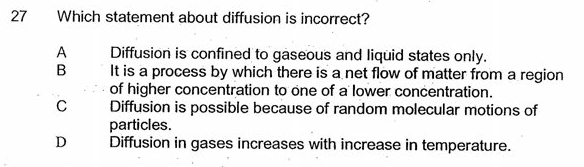Remarks: Why is the answer A? I thought all of the statements are correct.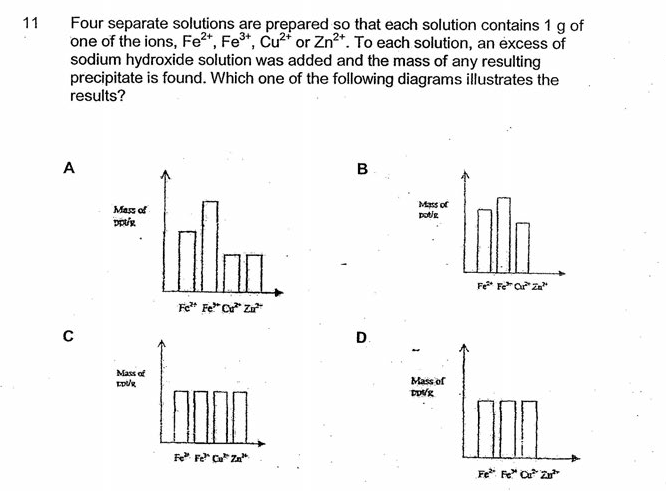Remarks: The answer is B. However, how do I know that the [Fe3+]>[Fe2+], given that the question did not say that the precipitates were left to stand, thereby allowing for oxidation of Fe2+ to take place?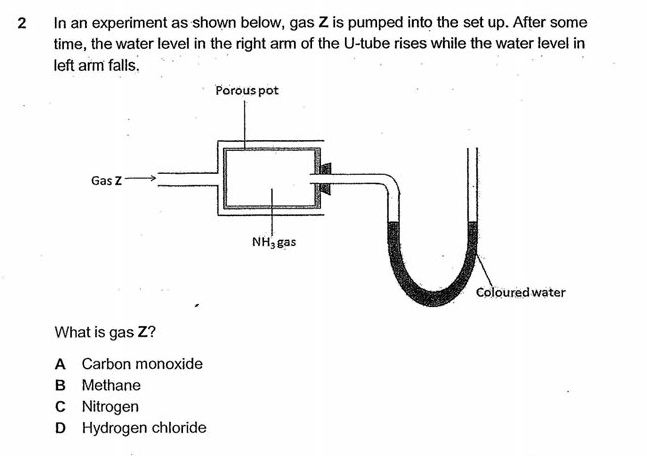My postulation: The change in water level would mean that the gaseous pressure has increased. The answer can’t be D because ammonium chloride would be formed (so presumably the gaseous pressure exerted on the LHS would decrease since an ionic salt is formed). However, what will happen when CO or N is pumped into the set up? Am I right in saying that the answer is B because methane does not react with ammonia?

• Originally posted by gohby:

I have a couple of O Level Science (Chemistry) MCQs on hand - would greatly appreciate if anyone could shed some light on these questions - cheers! :)Remarks: Why is the answer A? I thought all of the statements are correct.Remarks: The answer is B. However, how do I know that the [Fe3+]>[Fe2+], given that the question did not say that the precipitates were left to stand, thereby allowing for oxidation of Fe2+ to take place?My postulation: The change in water level would mean that the gaseous pressure has increased. The answer canâ€™t be D because ammonium chloride would be formed (so presumably the gaseous pressure exerted on the LHS would decrease since an ionic salt is formed). However, what will happen when CO or N is pumped into the set up? Am I right in saying that the answer is B because methane does not react with ammonia?

Q1. Diffusion also occurs in the solid and aqueous states.

Q2. Fe(OH)3 has a higher molar mass than Fe(OH)2.

Q3. Only CH4 has a lower molar mass than NH3. At constant temperature, the rate at which gas particles diffuse is inversely proportional to their molar mass.
• Hi UltimaOnline,

Thank you for your help :)

For 1 - how does diffusion take place in solids when the atoms are arranged in fixed positions? As for 3 I agree with what you mentioned, but how does it relate to the question?

• Originally posted by gohby:

Hi UltimaOnline,

Thank you for your help :)

For 1 - how does diffusion take place in solids when the atoms are arranged in fixed positions? As for 3 I agree with what you mentioned, but how does it relate to the question?

Hi Gohby, no prob, as always :)

Q3. CH4 (being lighter) diffuses into the porous container faster than NH3 (being heavier) can diffuse out, resulting in an increase in gaseous pressure within the porous container.

Q1. This isn't taught at either O levels or A levels, but Truth is Truth, Chemistry is Chemistry, and diffusion *does* occur in solids (the thermodynamic motivation for diffusion, is of course, thermodynamically favorable positive entropy change, which is also the thermodynamic motivation for Le Chatelier's principle).

https://en.wikipedia.org/wiki/Mass_diffusivity#Solids
https://en.wikipedia.org/wiki/Atomic_diffusion
https://en.wikipedia.org/wiki/Effective_diffusion_coefficient
https://en.wikipedia.org/wiki/Lattice_diffusion_coefficient
https://en.wikipedia.org/wiki/Kirkendall_effect
• Hello what are general tips you can give for mcq? what if i am unsure which is the answer?

Hello what are general tips you can give for mcq?

what if i am unsure which is the answer?

Eliminate whichever options you can eliminate, then make an educated guess for the remaining options, worse case scenario if you can't decide between remaining options, flip a coin, just don't leave it blank. But skip any qns you don't know, and get back to it after you've completed the paper. Don't waste time being stuck on any single qn.
• Hi UltimaOnline,

I would like to seek your opinion/concurrence for some of the questions where my answers diverged from the answers in the answer scheme :) :Remarks: The answer is B but I got A. Given the number of moles of ammonium nitrate used is 0.1, the number of moles of ammonia produced should be 0.1 too, so I think the volume produced should be 2.4dm³. Btw, what is the role of the aluminium powder in the reaction?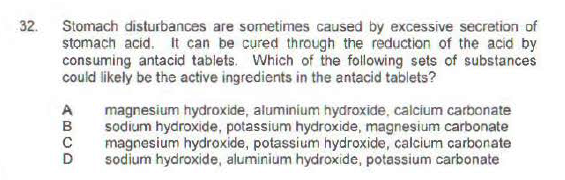Remarks: The answer is A. What should I be looking out for?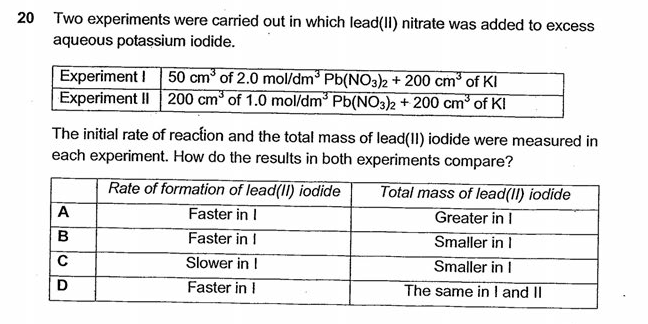Remarks: The answer is A but I think the answer is B. As greater number of moles of lead (II) nitrate was present in Experiment II , there should be more lead (II) iodide formed in experiment 2, thus the total mass of lead (II) iodide is smaller in I.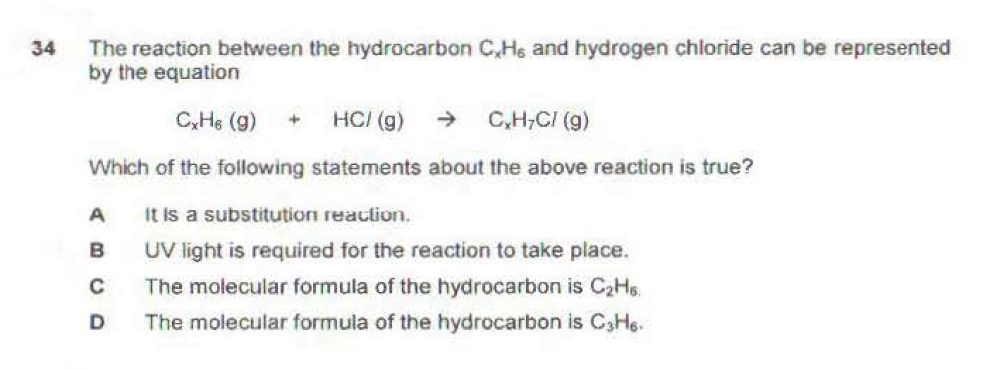Remarks:The answer is B but I think the answer is D. I would think this is an electrophilic addition reaction of an alkene rather than an FRS reaction.

• Originally posted by gohby:

Hi UltimaOnline,

I would like to seek your opinion/concurrence for some of the questions where my answers diverged from the answers in the answer scheme :) :Remarks: The answer is B but I got A. Given the number of moles of ammonium nitrate used is 0.1, the number of moles of ammonia produced should be 0.1 too, so I think the volume produced should be 2.4dmÂ³. Btw, what is the role of the aluminium powder in the reaction?Remarks: The answer is A. What should I be looking out for?Remarks: The answer is A but I think the answer is B. As greater number of moles of lead (II) nitrate was present in Experiment II , there should be more lead (II) iodide formed in experiment 2, thus the total mass of lead (II) iodide is smaller in I.Remarks:The answer is B but I think the answer is D. I would think this is an electrophilic addition reaction of an alkene rather than an FRS reaction.

Hi Gohby,

Your own answer for the last question about electrophilic addition of hydrogen halide across an alkene double bond is correct. Don't put too much trust in Singapore secondary schools' teachers' answers, or Singapore assessment practice books' answers, or Singapore TYS publishers' answers.

Q1. Al is oxidized to Al3+, and NO3- (OS of N = +5) is reduced to NH4+ (OS of N = -3). Thus a total of 0.1 + 0.1 = 0.2 mol of NH4+ is deprotonated by the OH- to generate 0.2 mol of NH3, which works out to be 4.8 dm3.

Q2. You need to use insoluble bases (ie. hydroxides and carbonates), as soluble alkalis are corrosive and will damage your mouth, throat and esophagus before it can reach your stomach to reduce acidity. (Medical note : aluminium is toxic and causes brain damage, thus shouldn't be used in drugs or additives, yet its use continues to be widespread, contributing to Alzheimer's disease and other related dementia medical conditions)

Q3. Either A or B could be correct, depending on the molarity of KI. If the molarity of KI is sufficiently low, it's *possible* for the mass of ppt generated in experiment 2 to be *less* than in experiment 1 (despite the moles of Pb2+ in experiment 2 being more than in experiment 1), because the total volume of solution in experiment 2 is greater. The secondary school teacher who set this question was being a smart-ass and must have been proudly confident of having set the trickiest O level Chemistry prelim paper question among all the secondary schools for that year (there's always an informal competition to set the toughest prelim papers, just look at the top JCs' prelim papers, most JC teachers themselves wouldn't be able to get an A grade if they sat for the top JCs' prelim papers within the allocated time). Unfortunately, he/she didn't realize that since the molarity of KI wasn't specified, if the molarity was sufficiently high, then even with a larger volume of solution, 99.9% of the moles PbI2(s) could be precipitated out in both experiments, then *your* answer would be correct. So this question has insufficient data to conclusively rule out either A or B.